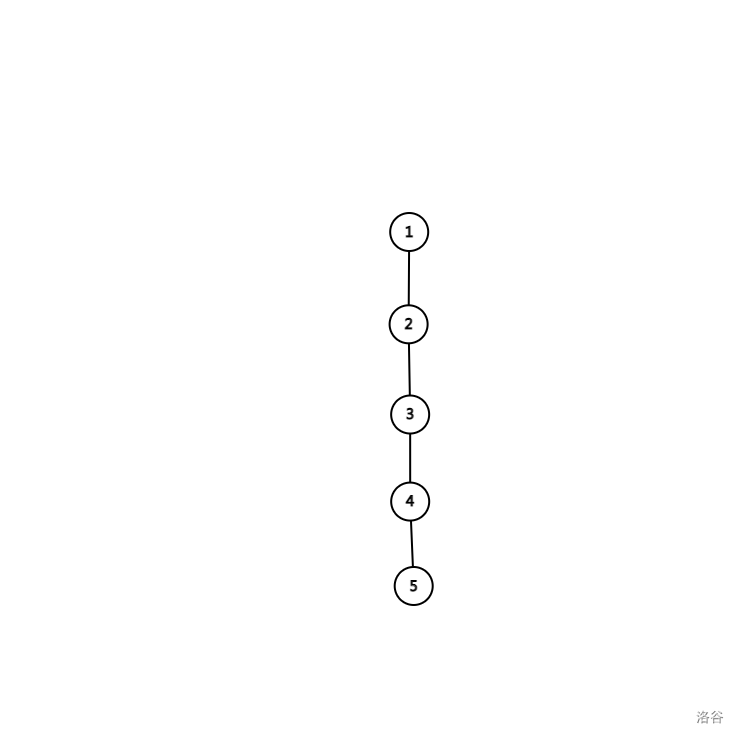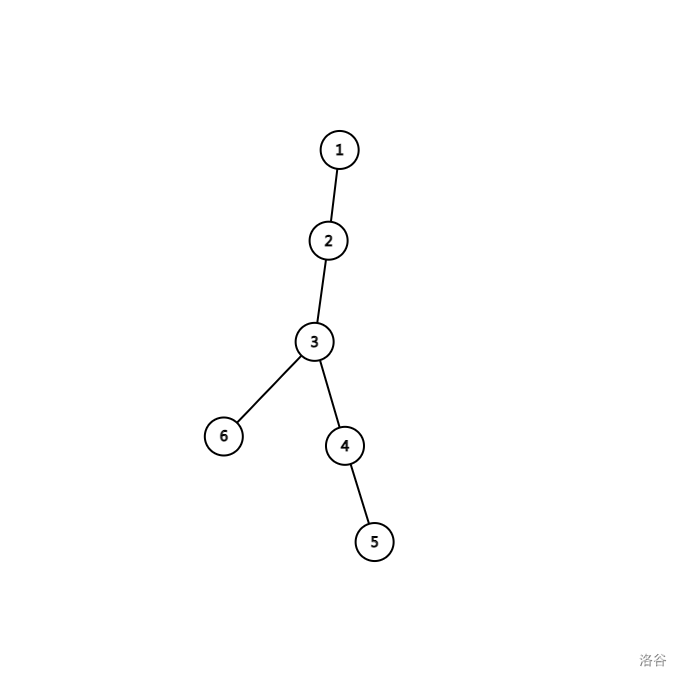## T1

### 题面描述

$$n$$ 块冰霜石和 $$n$$ 块猩红石，给出 $$m$$ 个不组合关系 $$(i, j)$$ ，表示第 $$i$$ 个猩红石和第 $$j$$ 个冰霜石不能组合在一起。现在你希望将冰霜石和猩红石配对，以发挥他们的最大效用，求最多可以将几对冰霜石和猩红石配对成功？

### 思路

Cms大佬说可以拿网络流骗分呢！

• 如果猩红石 $$i$$ 满足 a[i].size() $$= n$$ ，就是说它和所有的冰霜石都不能组合，答案一定是 $$n - 1$$ 。因为剩下的不组合关系不超过 $$n - 1$$ 个，不可能再把另一块猩红石堵死。
• 如果存在两块猩红石 $$i,j$$，满足 a[i].size() $$=$$ a[j].size() $$=n-1$$ ，并且 a[i]a[j] 完全相同，则说明满足 $$i$$ 和满足 $$j$$ 产生了冲突，必然要舍弃一个，答案也为 $$n - 1$$ ，因为剩下的不组合关系最多有一个，不可能再产生影响。
• 如果前两组情况都不存在，答案就是 $$n$$

## T3

### 题面描述

• 如果当前点是叶子节点，那么可以返回自己的任意一个与自己距离不超过 $$k$$ 的祖先。
• 如果当前节点不是叶子节点，那么可以访问自己的任意一个儿子节点。

### 思路